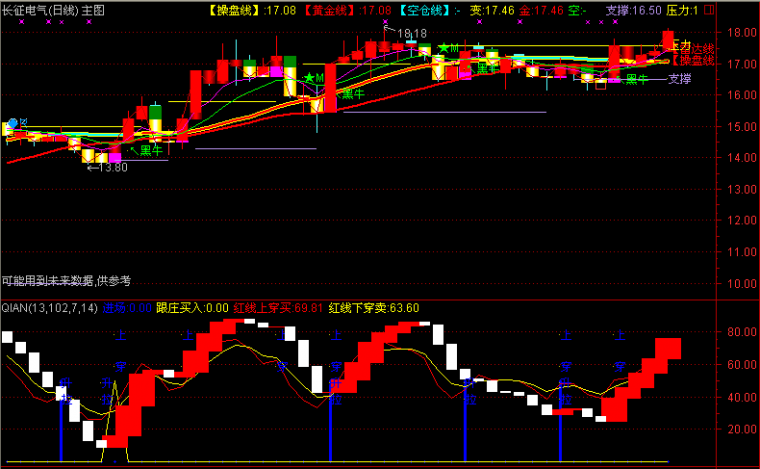﻿ 准备涨停预警超准指标公式-大智慧公式 －程序化交易（CXH99.COM）

# 准备涨停预警超准指标公式[大智慧公式]

R1赋值:150*以收盘价*0.95计算的获利盘比例

VAR3赋值:100*(收盘价-100日内最低价的最低值)/(100日内最高价的最高值-100日内最低价的最低值)的5日指数移动平均

VAR4赋值:(收盘价-126日内收盘价的最低值)/(126日内收盘价的最高值-126日内收盘价的最低值)*100

VAR5赋值:收盘价的2日指数移动平均-收盘价的120日指数移动平均

VAR6赋值:VAR5的68日指数移动平均

VAR7赋值:(VAR5+VAR6)

Y赋值:警的6日简单移动平均

Y1赋值:警-Y

G赋值:收盘价>=1日前的收盘价*1.08*100+0.5的整数部分/100

G1赋值:G*核心/5

RSV赋值:(收盘价-9日内最低价的最低值)/(9日内最高价的最高值-9日内最低价的最低值)*100

K赋值:(RSV的3日[1日权重]移动平均)

D赋值:(K的3日[1日权重]移动平均)

KK赋值:RSV的3日[1日权重]移动平均的整数部分

DD赋值:K的3日[1日权重]移动平均的整数部分

N赋值:KK+DD

R1:=150*WINNER(CLOSE*0.95);
VAR3:=EMA(100*(CLOSE-LLV(LOW,100))/(HHV(HIGH,100)-LLV(LOW,100)),5);
VAR4:=(CLOSE-LLV(CLOSE,126))/(HHV(CLOSE,126)-LLV(CLOSE,126))*100;
VAR5:=EMA(CLOSE,2)-EMA(CLOSE,120);
VAR6:=EMA(VAR5,68);
VAR7:=(VAR5+VAR6);

Y:=MA(警,6);
Y1:=警-Y;
G:=C>=INTPART(REF(C ,1 )*1.08*100+0.5)/100;
G1:=G*核心/5;
RSV:=(CLOSE-LLV(LOW,9))/(HHV(HIGH,9)-LLV(LOW,9))*100;
K:=(SMA(RSV,3,1));
D:=(SMA(K,3,1));
KK:=INTPART(SMA(RSV,3,1));
DD:=INTPART(SMA(K,3,1));
N:=KK+DD;(注：由于人数限制，QQ或微信请选择方便的一个联系我们就行，加好友时请简单备注下您的需求，否则无法通过。谢谢您！)

【字体： 】【打印文章】【查看评论

没有相关内容